# Question & Answer: Use the Programming Language —- > MATLAB…..

Use the Programming Language —- > MATLAB <—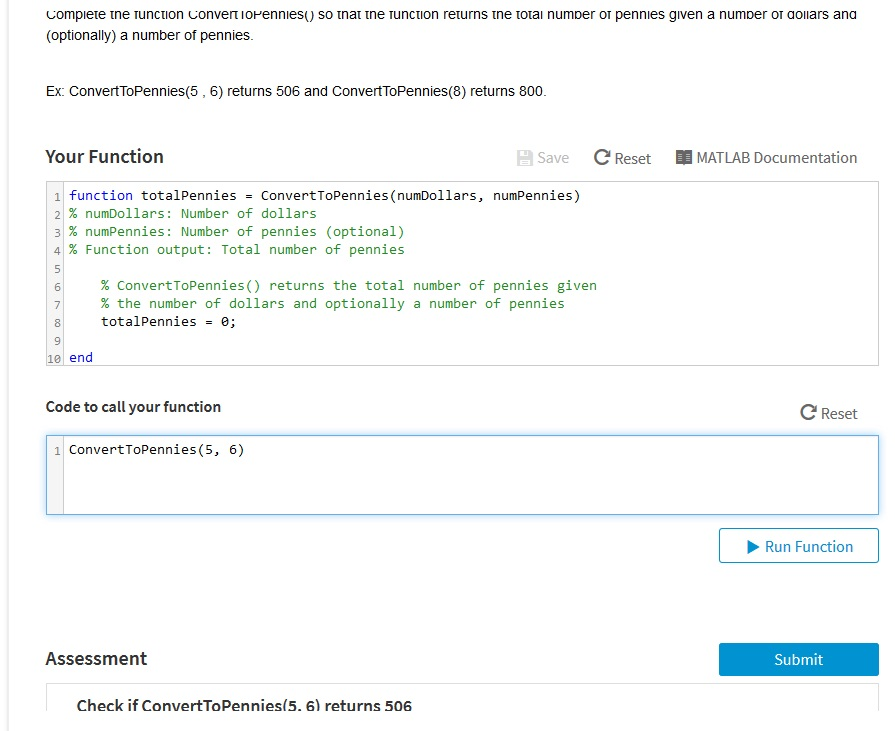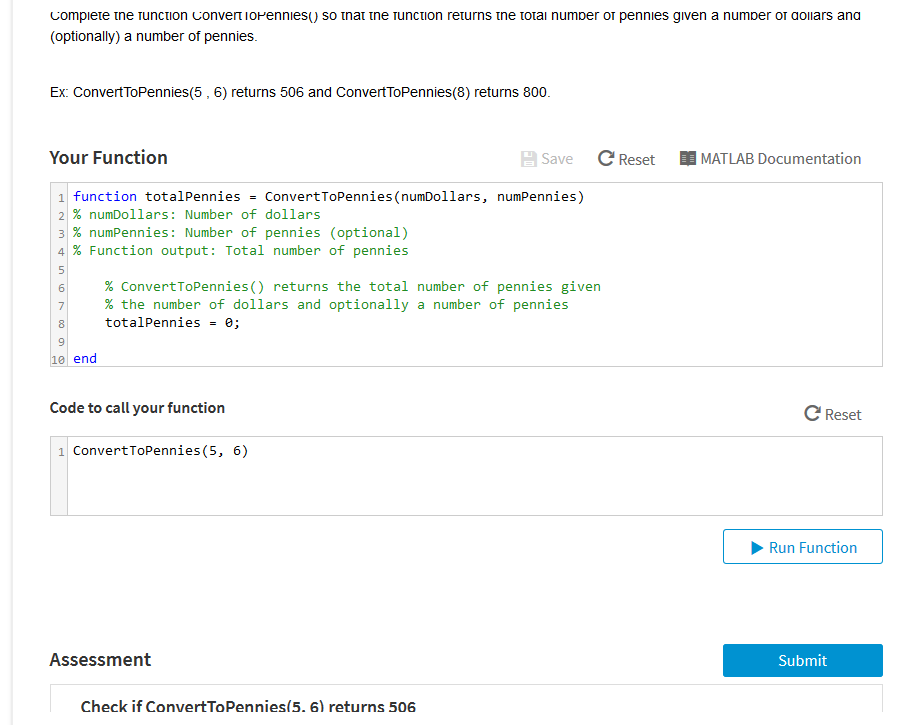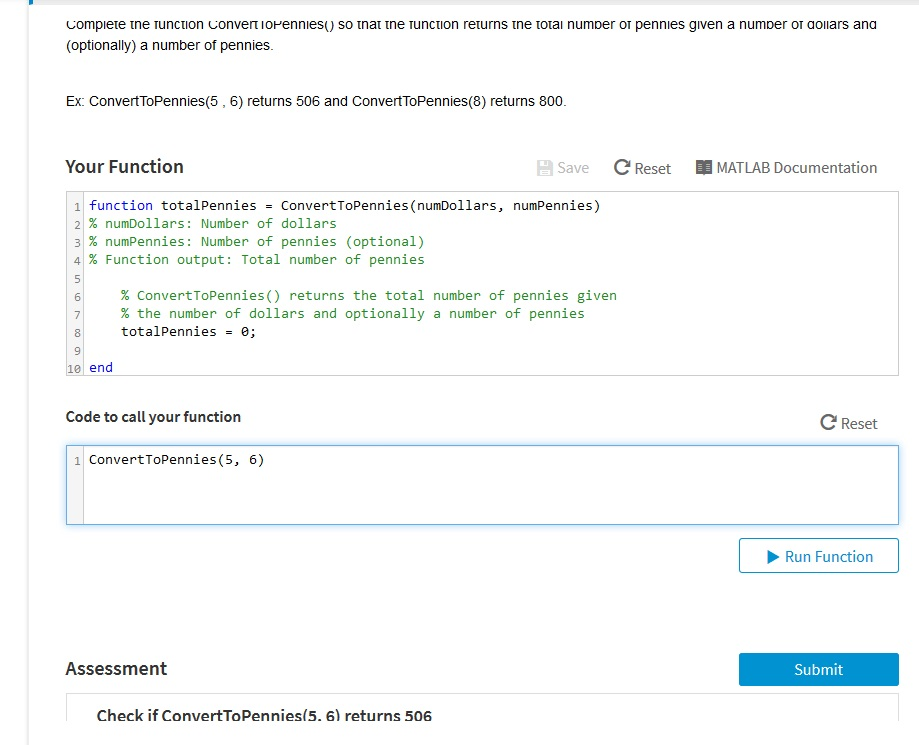Don't use plagiarized sources. Get Your Custom Essay on
Question & Answer: Use the Programming Language —- > MATLAB…..
GET AN ESSAY WRITTEN FOR YOU FROM AS LOW AS \$13/PAGE

totai numoer or pennies given a complete tne runction ConvertioPennies) so tnat tne runction returns tne (optionally) a number of pennies. numper or aoliars ana Ex: ConvertToPennies (5, 6) returns 506 and ConvertToPennies(8) returns 800. Your Function Save C Reset E MATLAB Documentation function tot al Pennies = ConvertToPennies(numDollars, numPennies) 21% numDollars: Number of dollars 31% numPennies: Number of pennies (optional) 41% Function output: Total number of pennies 5 % convert ToPennies() returns the total number of pennies given % the number of dollars and optionally a number of pennies 8 totalPennies 9 10 end Code to call your function C Reset 1 ConvertToPennies(5, 6) Run Function Assessment Submit Check if ConvertToPennies(5. 6) returns 506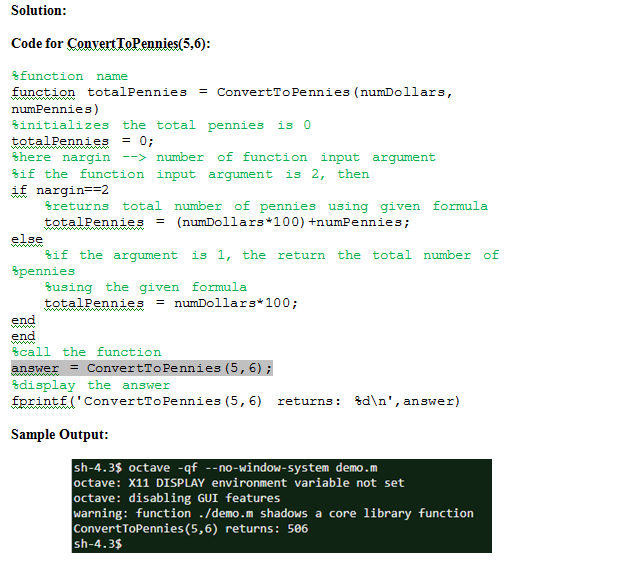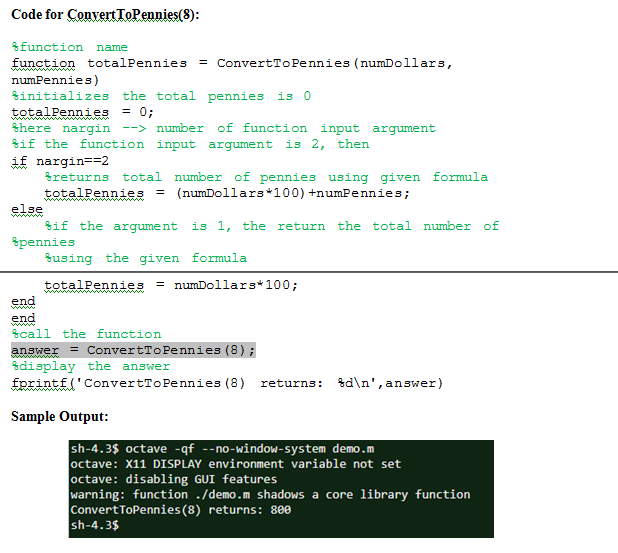Copyable Code:

Code for ConvertToPennies(5,6):

%function name
function totalPennies = ConvertToPennies(numDollars, numPennies)
%initializes the total pennies is 0
totalPennies = 0;
%here nargin –> number of function input argument
%if the function input argument is 2, then
if nargin==2
%returns total number of pennies using given formula
totalPennies = (numDollars*100)+numPennies;
else
%if the argument is 1, the return the total number of pennies
%using the given formula
totalPennies = numDollars*100;
end
end
%call the function